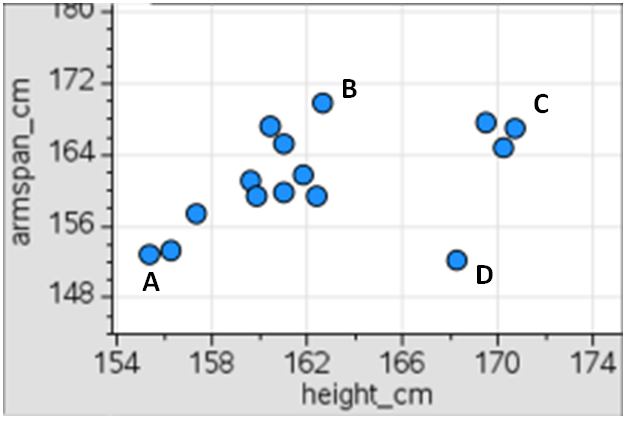# Statistics and Probability / Modeling Linear Relationships

Activity 23 of 24
In this lesson, students investigate patterns of association in bivariate data looking for direction, strength, and form of the relationship between the two variables.

## Planning and Resources

Objectives
Students identify an association between two quantitative variables as strong or weak, positive or negative, and interpret the association in context. They model relationships between two quantitative variables with linear equations and assess the fit of the model using the sum of the absolute value of the deviations.

Vocabulary
association
linear
regression
rate of change
residual

Standard:

## Lesson Snapshot

#### Understanding

A model that "fits" the data well minimizes the sum of the absolute deviations from line.

### What to look for

To analyze how well a linear equation "fits" the data, construct a scatter plot of the residuals for any patterns or predictability. Models that result in a pattern in the residual plot do not model the data well.

### Sample Assessment

The scatter plot below shows the relationship between height, in centimeters, and the arm span, in centimeters, of 15 students in a class.Based on the scatter plot, determine which ordered pair would be the farthest from the best fit line.

a) A
b) B
c) C
d) D